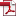• KSII Transactions on Internet and Information Systems
Monthly Online Journal (eISSN: 1976-7277)

# A Rapid Convergent Max-SINR Algorithm for Interference Alignment Based on Principle Direction Search

Vol. 9, No. 5, May 30, 201510.3837/tiis.2015.05.012, Download Paper (Free):#### Abstract

The maximal signal-to-interference-plus-noise ratio (Max-SINR) algorithm for interference alignment (IA) has received considerable attention for its high sum rate achievement in the multiple-input multiple-output (MIMO) interference channel. However, its complexity may increase dramatically when the number of users approaches the IA feasibility bound, and the number of iterations and computational time may become unacceptable. In this paper, we study the properties of the Max-SINR algorithm thoroughly by presenting theoretical insight into the algorithm and by providing the potential of reducing the overall computational cost. Furthermore, a novel IA algorithm based on the principle direction search is proposed, which can converge more rapidly than the conventional Max-SINR method. In the proposed algorithm, it searches along the principle direction, which is found to approximately point to the convergence values, and can approach the convergence solutions rapidly. In addition, the closed-form solution of the optimal step size can be formulated in the sense of minimal interference leakage. Simulation results demonstrate that the proposed algorithm outperforms the conventional minimal interference leakage and Max-SINR algorithms in terms of the convergence rate while guaranteeing the high throughput of IA networks.